Next: The Gradient-Based Approach Up: Finding Optic Flow Previous: Finding Optic Flow

The Feature-Based Approach

The method of finding image displacements which is easiest to understand is the feature-based approach. This finds features (for example, image edges, corners, and other structures well localized in two dimensions) and tracks these as they move from frame to frame. This involves two stages. Firstly, the features are found in two or more consecutive images. The act of feature extraction, if done well, will both reduce the amount of information to be processed (and so reduce the workload), and also go some way towards obtaining a higher level of understanding of the scene, by its very nature of eliminating the unimportant parts. Secondly, these features are matched between the frames. In the simplest and commonest case, two frames are used and two sets of features are matched to give a single set of motion vectors. Alternatively, the features in one frame can be used as seed points at which to use other methods (for example, gradient-based methods -- see the following section) to find the flow.

The two stages of feature-based flow estimation each have their own problems. The feature detection stage requires features to be located accurately and reliably. This has proved to be a non-trivial task, and much work has been carried out on feature detectors; see the reviews in Sections 3 and 4. If a human is shown, instead of the original image sequence, a sequence of the detected features (drawn onto an empty image), then a smoothly moving set of features should be observable, with little feature flicker. The feature matching stage has the well known correspondence problem of ambiguous potential matches occurring; unless image displacement is known to be smaller than the distance between features, some method must be found to choose between different potential matches.

Finding optic flow using edges has the advantage (over using two dimensional features) that edge detection theory is well advanced, compared with that of two dimensional feature detection. It has the advantage over approaches which attempt to find flow everywhere in the image, such as the method developed by Horn and Schunk in  (see Section 1.2), that these other methods are poorly conditioned except at image edges; thus it is sensible to only find flow at the edges in the first place.

Most edge detection methods which have been used in the past for finding optic flow are related to either finding maxima in the first image derivative, see , or finding zero-crossings in the Laplacian of a Gaussian of the image, see . The major problem with using edges is that unless assumptions about the nature of the flow are made, and further processing (after the matching) is performed, only the component of flow perpendicular to each edge element can be found. Unfortunately, the assumptions made will invariably lead to inaccuracies in the estimated flow, particularly at motion boundaries, etc.

The first major work taking this approach was that of Hildreth; see . The edges are found using a Laplacian (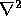) of a Gaussian edge detector, and the image motion is found at these edges by using the brightness change constraint equation (see the following section). Various additional constraints necessary to recover the motion parallel to the edge direction are discussed. The constraints all make the basic assumption that the image flow is smoothly varying across the image. Thus each minimizes some measure of flow variation. The measure chosen is the variation in velocity along the edge contours. If this is completely minimized, the data is largely ignored, so a balancing term is added to the minimization equation which gives a measure of the fit of the estimated flow to the data. Thus the following expression is minimized;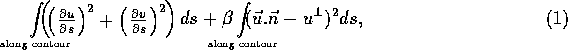where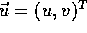is the estimated optic flow,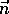is the unit vector perpendicular to the edge,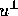is the measured component of flow perpendicular to the edge, s is the ``arclength'' parameter and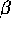is the weighting factor which determines the relative importance of the two terms. The first term expresses the variation in estimated flow, and the second term expresses the quality of the fit of the estimated flow to the measured edge displacements. The expression is minimized using the conjugate gradient approach, also described in . The overall algorithm is intrinsically sequential, and is fairly computationally expensive.

The use of edges not just in space but in space-time was introduced by Buxton and Buxton; the theory is developed in , and testing is reported in . Here they use the d'Alembertian (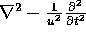) of a spatiotemporal Gaussian, instead of the Laplacian. The velocity parameter u and the scale of the Gaussian filter need to be applied at different channels to search for different motion possibilities. Although noise suppression was claimed to be greater than that achieved using Hildreth's approach due to the extended dimensionality of the filter, a corresponding increase in computational expense also resulted. In  this work is extended; the negative metric is replaced with the positive one (i.e.\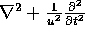) to reduce noise sensitivity and removing ``curious'' cross-over effects (mentioned in , but never utilized). In , instead of using Hildreth's velocity smoothness constraint, an object's motion and position parameters are estimated directly from the measurements of the normal components of flow. However, this ``3D solution to the aperture problem'', solved with a least squares fit to the normal flow, assumes that the objects in question are planar, and that the image is already segmented into differently moving objects.

In  Castelow et. al. use Canny edgels (edge elements found using the Canny edge detector) to find optic flow at edges. In this method, developed originally by Scott (see ), the flow parallel to the edges is recovered by iterative relaxation of the edgel matching surface; the edgels near the high curvature parts of the contours can have both components of flow found from the data, so these cues to the full flow are propagated along the contour into less highly curved sections by relaxation. Thus local support of optic flow is used to improve the motion estimate iteratively. The results presented did not appear to be as good as those shown in  and , although the method has been successfully used by Murray et. al. to reconstruct three dimensional objects in structure from motion work; see  and . This method of finding optic flow has also been used to track cataracts, in .

In , Gong and Brady found the full flow at points of high curvature on zero-crossing contours using their ``curve motion constraint equation''. The flow is then propagated along the contours by using a wave/diffusion process. This method was developed further by Wang et. al. in . Edges are found using Canny's edge detector, and are then segmented and grouped. ``Corners'' in the edges are found by fitting local B-splines, and the edges at these points are matched from one frame to the next (using a cross-correlation method to achieve correct matches) to give the full flow. This flow is then propagated along the edges using the wave/diffusion process. The algorithm was implemented in parallel, and so achieved an impressive speed compared with the original algorithm, and compared with Hildreth's approach. The results obtained were good (for an edge-based method), although the full flow was not always correctly estimated along straight portions of edges. The use of high curvature parts of edges as two dimensional features can lead to the rejection of features which are well localized in two dimensions (and therefore useful for recovery of optic flow) but are not placed on well defined edges. ASSET [103,102,100] finds two dimensional features directly from the image, and not via an intermediate edge finding stage, and therefore avoids this problem.

Some of the ways in which edges have been used to find optic flow for different purposes are now given.

In  Deriche and Faugeras track straight edges in two dimensions using Kalman filters for each edge. The edges are found using the Deriche filter (see ) and are represented by their length, orientation and position. The resulting matched and tracked line segments are fed into a structure from motion algorithm; however, this approach is limited to fairly structured environments, as it only uses straight lines.

In  Bolles et. al. use spatiotemporal edge filters as developed by Buxton and Buxton (see earlier) to track features in single raster lines over time. The tracks of features within these raster lines create ``epipolar-plane images''; these contain information about the three dimensional structure of the world. The assumption is made that the images are taken in quick succession compared with the motion of the features, so that there is no correspondence problem. In  Tomasi and Kanade track edges found using the Laplacian of a Gaussian in an approach very similar to that of Bolles. From the tracked edges the shape of an object is computed ``without first computing depth''. Very accurate results are obtained, using highly constrained camera motion.

In  Sandini and Tistarelli use Laplacian of a Gaussian edge contours, tracked over time by a camera moving with constrained motion. The tracked contours are used to construct the three dimensional structure of objects in view. In  Blake and Cipolla (again, given a deliberate, constrained and known camera motion) use the deformation of edge contours to recover surface curvature in the world. In  Faugeras discusses the problems of tracking image edges associated with non-rigid bodies. In  Chen and Huang match straight line segments in stereo sequences for object motion estimation.

Optic flow methods based on the detection of two dimensional features (which shall be known as corners for the purpose of this discussion) have the advantage that the full optic flow is known at every measurement position. The (related) disadvantage is that only a sparse set of measurements is available, compared with methods which give a measurement at every image position. However, if the features are found appropriately then the maximum possible number of different optic flow measurements in the image should be recoverable, without the degradation in flow estimate quality introduced by the various assumptions and constraints required to recover the full flow using other methods. Note that arguments have often been made that gradient-based (and related) methods have their flow estimations achieving the most well conditioned results near edges. In the same way, flow estimates found at edges are most accurate near corners. In the ASSET [103,102,100] system, corners are used for finding optic flow, and image segmentation is based on this; the segmentation results are then further refined by using ``intra-frame'' image edges. This appears to be a sensible use of the image data, since the flow is found at those places where this can be most accurately achieved, and remaining (instantaneous) image information about possible object boundaries can be used to make adjustments to the interpretation made using the flow. Another advantage of using corners is that the early data reduction achieved should allow computationally efficient algorithms to be developed.

Some of the published discussions on optic flow found from moving corners and some of the ways in which this information has been recovered and used are now described.

Much work on structure from motion has assumed that the flow field is only recovered at relatively sparse image positions. The work has largely consisted of theory relating how much scene information can be given from different numbers of views of varying numbers of ``world points''. For example, in  Faugeras and Maybank show that in general the least number of point matches (found using two viewpoints) which allows only a finite number of interpretations of the camera positions (relative to the world) is five. With this number of matches, there are a maximum of ten possible interpretations of the camera's motion.

With regard to the problem of matching corners from one frame to the next, early work was undertaken by Barnard and Thompson; see . Here the Moravec interest operator is used to find points which are well localized in two dimensions in consecutive images. Next, the (computationally expensive) method of iterative relaxation of the matching surface is used to find the optimal set of point matches. Each point in the first frame is given a probability of matching to each point in the second; the initial probability is inversely proportional to the sum of the squares of the difference between the brightness of each point within a small patch centred on the ``corner'' in the first frame and a small patch centred on the ``corner'' in the second. Then relaxation is applied to force the flow field to vary smoothly. (This matching validation approach could be overly restrictive in certain situations, where flow discontinuities are important sources of information.) In  this method is used to successfully detect moving objects as seen by a moving camera; this work is discussed later.

In  Charnley and Blissett describe the vision system DROID. DROID uses two dimensional features found with the Plessey feature detector (see Section 4), and tracks these over many frames in three dimensions using a Kalman filter for each feature. Time-symmetric matching is performed on the basis of feature attributes, using (where available) predicted feature positions from the filters. The tracked features are used to construct a three dimensional surface representing the world. The constraint is applied that the world must contain no moving objects.

In  L.S. Shapiro et. al. describe a corner tracker using the Wang corner detector (see ). Small inter-frame motion is assumed. Corners are matched using correlation of small patches. Where no matches are found, motion is predicted, using again the correlation of small patches, in a manner very similar to the method of Lawton (see the following paragraph). Tracking is achieved over many frames using simple two dimensional filters which model image velocity and (if required) image acceleration.

In  Lawton uses optic flow to recover world structure assuming a static environment and translational camera motion. Image flow is found by taking corners from one frame only, and using correlation of small patches centred at the features with patches placed at all possible positions in the second frame. Corners are found by using an approach similar to that of Moravec, (see Section 4) but are only looked for along Laplacian of Gaussian edges. The flow is found to sub-pixel accuracy by using inter-pixel interpolation in both frames, once the approximate displacement has been found. Again, cross-correlation is used for this. In  Adiv uses Lawton's method to find the structure and motion of independently moving planar objects; the planar spatial regions are later fused into multi-facet objects.

In  Sinclair et. al. use the motion of corners to find three dimensional planes in the world (via projective invariants) and also the direction of the camera's motion. Burger and Bhanu, in  use the motion of ``endpoints and corners of lines as well as centers of small closed regions'' to find the direction of translation of the camera. Work on image segmentation using the motion of two dimensional features includes , ,  and .

Some work has been carried out which attempts to integrate the motion of corners and edges. In  Faugeras et. al. compare recovered three dimensional structure from the motion of points and edges theoretically and experimentally. In  Stephens and Harris used edges and corners to recover the three dimensional structure of a static environment (in a project related to the DROID system). They integrated the tracked features with edges in an attempt to provide wire-frame models of static objects in the world. This integration proved difficult, and was dropped in later versions of DROID, where edges were not used.

The details of how ASSET recovers a useful image flow field from sets of two dimensional features are given in [103,102,100], where some justification of the approach taken is also given.Next: The Gradient-Based Approach Up: Finding Optic Flow Previous: Finding Optic Flow

© 1997 Stephen M Smith. LaTeX2HTML conversion by Steve Smith (steve@fmrib.ox.ac.uk)